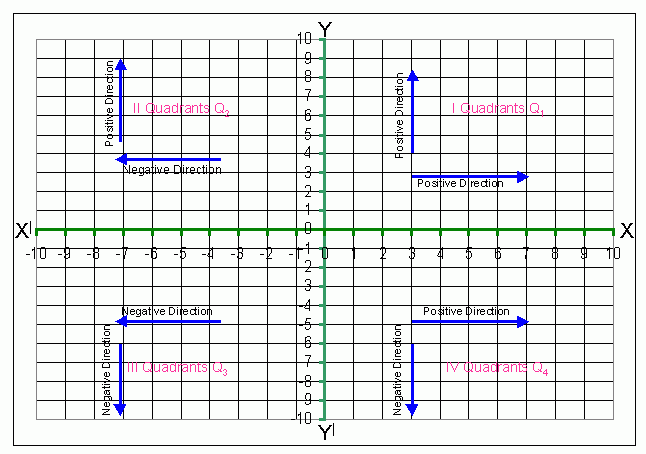Name: ___________________Date:___________________In the above diagram, X and Y axes divided the plane into four regions and these regions are called Quadrants and they are numbered Q1, Q2, Q3 and Q4, in the anti clock wise direction the first quadrant is denoted as Q1, the second quadrant is denoted as Q2, the third quadrant as Q3 and the fourth quadrant as Q4. The right hand side towards east form the Y-axis is considered as positive direction. The left hand side towards west from the Y-axis is considered as negative directions. The upward direction towards north from X-axis is positive direction and the downward side towards the south is negative direction. In the first quadrant Q1 the coordinates x and y are (+,+). That is a positive real number and a positive real number. In the second quadrant Q2 the coordinates x and y are (-,+). That is a negative real number and a positive real number. In the third quadrant Q3 the coordinates x and y are (-,-). That is a negative real number and a negative real number. In the first quadrant Q4 the coordinates x and y are (+,-). That is a positive real number and a negative real number.